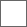• 2回复贴，共1

### 【讨论】几种方法证明a^n-b^n=。。。

Sn=x1+x2+x3...+xn
=(1-x^n)/(1-x)
1-x^n=(1-x)*Sn
=(1-x)*(1+x+x^2+x^3...+x^(n-1))

#### 扫二维码下载贴吧客户端